Next: The Next Bit Theorem Up: Pseudo-random number generators Previous: Pseudo-random number generators   Contents

Let, whereare primes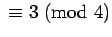. For each prime,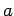is a square if and only if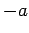is not a square (Lemma 22). This implies that, if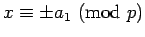and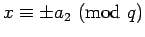, there will be exactly one choice which makes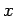a square mod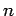. Hence, if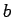is a square mod, exactly one of its four square roots will also be a square. This principal square root will be denoted by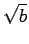.

The quadratic generator uses a randomly chosen square(called the seed) not divisible by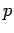or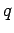to generate a sequence of 0's and 1's (bits). The sequence ismod 2, where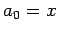and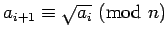: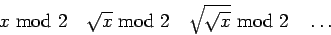(from a practical point of view, it is simpler to generate the sequence starting with the last number and squaring)

As a small example withand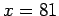, the sequence ofis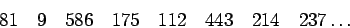(note that, not 3) which gives the sequence of bits 11010101.

Translated from LaTeX by Scott Sutherland
2002-12-14